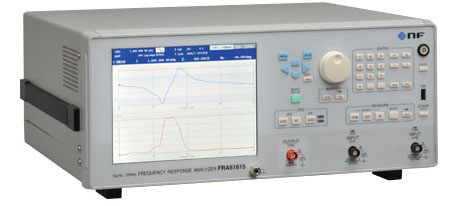# Impedance Measurement of Copper Wire by FRA

## Frequency Response Analyzer FRA51615, Bipolar DC Power Supply BP series

As energy conservation advances, transformers and motors are becoming more efficient. Improvement of efficiency requires reduction of loss (iron loss and copper loss). The parts used in the devices are operating at high frequency. Therefore, we should evaluate the copper wire used in the devices in a state close to the actual condition, that is, at high frequency.

By combining FRA (Frequency Response Analyzer) with Bipolar DC Power Supply operated in CC mode, high frequency impedance characteristics of copper wire can be measured.

### Key Features

• Constant current operation
• Measurement can be made with the AC constant current and the DC constant current (bias current) applied
• Frequency Response Analyzer FRA51615 can measure and display the impedance frequency characteristics

### Measurement System### Measurement Result Impedance/Phase Frequency Response of Different Diameter Wires### Measurement setup

• Measure the impedance of the copper wire at constant current by Frequency Response Analyzer FRA51615 and Bipolar DC Power Supply (BP4610).
• Measure the voltage across the copper wire and the current flowing through the copper wire using a shunt resistance (1 Ω), and graphically display the impedance frequency characteristics.
• The samples are the impedance of copper wires with different diameters. The current is sine wave 6 Apk, and the frequency range is 10 Hz – 70 kHz.

### Measurement result

• The Impedance is constant up to around 100 Hz, the influence due to frequency change is small, but it is considered that the reactance is influencing beyond around 1 kHz.
• The impedance changes of copper wire of 3.3mm is different compared to others. The wiring shape may be affecting.

### Measuring Equipment

#### Frequency Response Analyzer FRA51615Suitable for measuring servo stability, loop gain, impedance, and resonance frequency.

• Measurement Frequency: 10 µHz – 15 MHz
• Amplitude accuracy : ±0.01 dB
• Phase accuracy : ±0.06°
• Dynamic range : 140dB
• Isolation voltage : 600Vrms max.
• Automatic integration, amplitude compression, calculation function, impedance display function
• Bode diagram, Nyquist diagram, Nichols plot, Cole-Cole plot

#### Bipolar DC Power Supply BP seriesWide range bipolar power supply capable of constant current / constant voltage output

• Output voltage: ±60 V (120 Vp-p)
• Output current
BP4610: ±10 A
Available from ±20 A to ±100 A
• Frequency response
DC – 150 kHz maximum (CV mode)
DC – 70 kHz at maximum (CC mode)
• Voltage / current four quadrant output
• Built-in 255 steps sequential signal source